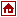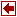MINOPT
A Modeling Language and Algorithmic Framework for Linear, Mixed-Integer, Nonlinear, Dynamic, and Mixed-Integer Nonlinear Optimization

 Authors: C. A. Schweiger Prof. C. A. Floudas

MINOPT was developed in the CASL laboratory in the Department of Chemical Engineering at Princeton University. It's development has been motivated by the need for efficient methods for solving Mixed-Integer Nonlinear Programming problems as well as handling numerous options associated with these problems. Further development was motivated by the need to handle dynamic models and solve problems involving both differential and algebraic constraints. The result is an advanced modeling language and algorithmic framework.

Description

MINOPT is a comprehensive, powerful, and flexible package for the solution of various types of optimization problems. It features both an advanced modeling language for the clear and concise representation of complex mathematical models as well as robust algorithmic framework for the efficient solution of wide variety of mathematical programming problems.

MINOPT features:

• Clear and concise representation of complex mathematical models
• Support for a broad variety of natural mathematical expressions
• Modeling: Algebraic and Dynamic
• Capability to add, change, or delete the sets, variables, data, and constraints easily
• Capability to accept model information and data provided in separate input files
• Checks of model syntax and consistency
• Efficient solution for Mixed-Integer Nonlinear Programming problems
• Efficient solution for problems with dynamic models
• Efficient integration and sensitivity analysis
• Connection to Chemkin for kinetic modeling
• Ability to switch easily among various solvers
• Ability to fine tune the solution algorithms with an extensive list of options
• Portable models which can be used across various platforms

MINOPT model types:

• Linear Programs (LP)
• Mixed Integer Linear Programs (MILP)
• NonLinear Programs (NLP)
• NLPs with Dynamic Models (NLP/DAE)
• Mixed Integer NonLinear Programs (MINLP)
• Dynamic Simulations
• MINLPs with Dynamic Models (MINLP/DAE)
• Optimal Control Problems (OCP)
• Mixed Integer Optimal Control Problems (MIOCP)

Reference